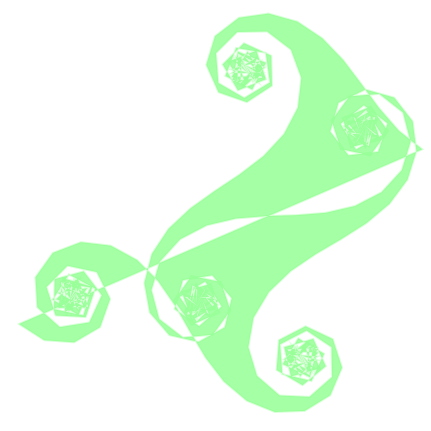## Tuesday, March 11, 2008

### NodeBox

I had attended to "Python Developer Camp 2008 Winter" held in Matsumoto(Japan) on the last weekend. I learned about NodeBox. NodeBox is a software on MacOSX. You can obtain it from NodeBox | Home.

Using NodeBox you can make images and movies from a small amount of python code. It is very fun. You can change the number literal by dragging it with command key. It is nice idea.`from math import sin, cosSIZE = 431size(SIZE, SIZE)x = 19y = 324th = 1dth = 0.5ddth = 0.1s = 30colormode(RGB, 1)stroke(color(0.5, 1, 0.5, 0.3))strokewidth(2)fill(color(0.5, 1, 0.5, 0.7))beginpath()moveto(x, y)for i in range(313):    x += sin(th) * s     y += cos(th) * s    th += dth    dth += ddth    lineto(x, y)    print x, yendpath()`